Science, Maths & Technology

### Become an OU student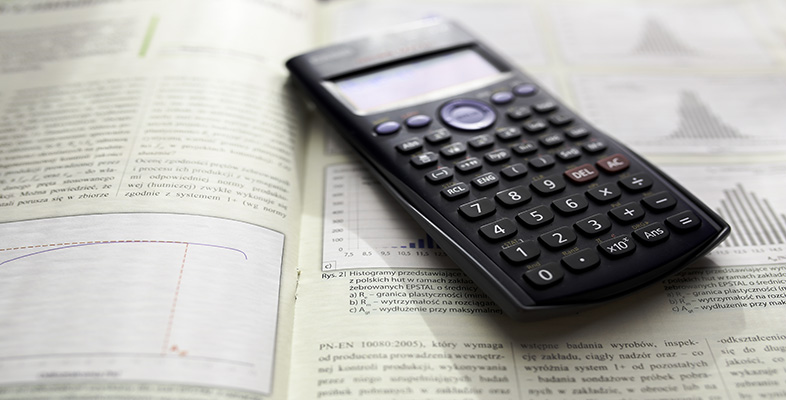Using a scientific calculator

Start this free course now. Just create an account and sign in. Enrol and complete the course for a free statement of participation or digital badge if available.

# 12.2 Common operations

To Key sequence
Turn on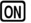Turn off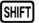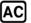(OFF)

Note that the calculator will automatically turn off if not used for about 6 minutes.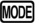(SETUP)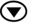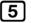(◄CONT►) then use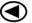and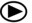to adjust, and presswhen finished.

Restore the factory settings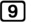(CLR)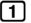(Setup)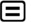(Yes)Clear the contents of all memories(CLR)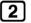(Memory)(Yes)Restore the default settings and clear all memories(CLR)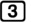(All)(Yes)Cancel a calculation, or exit menusObtain a decimal answer in Math modeToggle between exact and decimal answers in Math mode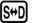Toggle between displaying fractions as improper fractions or mixed numbers in Math mode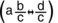Use the result of the previous calculation…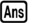… e.g. to find 42 minus the previous result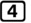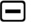Store a value in ‘M’ memory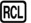(STO)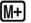(M)

Clear a value stored in the M memory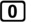(STO)(M)

Add a value to that in the ‘M’ memorySubtract a value from that in the ‘M’ memory(M-)

Display the value stored in the ‘M’ memory(M)

Use the value in the ‘M’ memory in a calculation…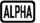… e.g. to find 42 minus the value in the ‘M’ memory(M)Set angles to be measured in degrees(SETUP)(Deg)

Set angles to be measured in radians(SETUP)(Rad)

Set answers to be displayed to 3 decimal places (To use a different number of decimal places, replacewith that number)(SETUP)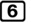(Fix)Set answers to be displayed in scientific notation with 3 significant figures (To use a different number of significant figures, replacewith that number)(SETUP)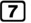(Sci)Cancel any display rounding settings(SETUP)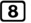(Norm)The A, B, C, D, X, Y memories are used similarly to the M memory, except that these have no ‘add to’ and ‘subtract from’ functions.